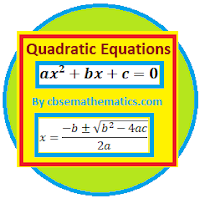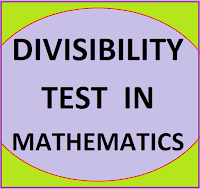## Posts

Showing posts from October, 2018

### Resource Centre Mathematics

Resource Centre Mathematics Mathematics worksheet, mathematics basic points and formulas, mathematics lesson plan, mathematics multiple choice questions Workplace Dashboard CBSE Syllabus For Session 2023-24 For  :   Classes IX & X    |   Classes XI & XII Watch Videos on Maths Solutions CLASS IX MATHEMATICS FORMULAS &  BASIC CONCEPTS

### CBSE Class 10 Maths Formulas Chapter-04 | Quadratic Equations### DIVISIBILITY TEST- CBSE MathematicsDIVISIBILITY TEST INMATHEMATICS Short cut method of checking the divisibility of numbers. Divisibility test for 2, 3, 4, 5, 6, 7, 8, 9, 10, 11 with examples and explanation

### Limits and Continuity-cbse mathematics